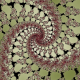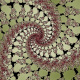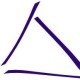## Manipulation of Implicit Functions (With an Eye on CAD)

Most people are familiar with the equation for a circle,$x^2+y^2 = r^2$:I prefer to think of it as the curve where$f(x,y) = \sqrt{x^2+y^2}-1$ is zero — the locations where the Euclidean distance from$(0,0)$ is one.This curve is called an implicit function, and when we look at it as the curve on which a function of two variables is zero, a lot of possibilities open up for manipulating it. Clearly we can translate it by adding or subtracting from$x$ and$y$:We can also scale them by$s$ like so:$s*\left(\sqrt{\left(\frac{x}{s}\right)^2+\left(\frac{y}{s}\right)^2}-1\right)$.   I’m intentionally preserving unit distance here, you will see why this is useful later.Beyond that, we can change our notion of distance to produce different shapes, by changing the powers used above. For example,$\sqrt{|x|^3+|y|^3}-1$:Or,$\sqrt{x^4+y^4}-1$:These notions of distance correspond to the$p$-norms. As you increase the power, these tend to the unit ball of the super norm,$\max(|x|,|y|)$:Now, consider what$|x| -1 = 0$ and$|y| -1 = 0$ would look like:$\max(|x|,|y|) - 1 = \max(|x| -1,|y|-1) = 0$ is the intersection. Maximum intersects things. Conversely, minimum unions things.Two things need to be clarified: what and why.

What are we unioning or intersecting? After all, we’re working with functions, not objects. However, we can look at the functions as a way of representing objects — we already have been, we just haven’t discussed it. The boundary is the$f(x,y) = 0$, negative regions are the interior and positive regions are the exterior. We can think of the function as representing the “outwardness” of the object.

Now why does it work? Sometimes, the best way to answer such a question is to look at a simple example. Let’s consider unioning and intersecting two simple one dimensional objects, line segments. We can look at the corresponding “outwardness” functions not as a colored background but height on the y-axis.(Technical comment, disregard unless you’re a very big nitpick: The ‘outwardness’ functions aren’t the normal ‘outwardness’ functions of line segments — they’re slices of a square — but they avoid certain questions I don’t want to deal with here.)

In short, if one of the functions is negative, the minimum will be negative and thus inside — being inside one means you’re inside the union. Similarly, if one of them is positive, the maximum will be positive — if you’re outside one, you’re outside the intersection.

One thing that would be really interesting to do is to make a rounded union or intersection. Consider the following union of two squares.The sharp right-angle transition in the curve$\min(x,y)=0$ corresponds to the interface between the two unioned objects.

If we could round the transition, we would get nice round unions. So we construct a rounded minimum function, replacing that corner with a quarter of a circle.$\mathrm{rmin}_r(a,b) = \left\{\begin{array}{cc} \min(a,b) & |a-b| \geq r \\ b + r*\sin(\pi/4+\mathrm{asin}(\frac{a-b}{r \sqrt{2}})) - r & |a-b| < r \\ \end{array} \right.$If we use this to union the squares, we get:(NB. This particular formation of rounded unions is a little bit naive. If you union and the sides aren’t orthogonal, this version will make a bump, which is not the expected behavior. This can be corrected for quite easily by looking at the dot product of the discrete gradients.)

Another neat thing we can do is make shells of the object. By this I mean that we create a new object that is a certain width from the edge of our original object. For example, this is the shell of width 0.2 of a square:We do this by taking the absolute value and then subtracting half the desired width. Taking the absolute value makes the interior into exterior and then subtracting ‘pushes out’ the boundary.

Again, we consider a one dimensional example. It is very similar to what happens to a slice down$y=0$ of the above shell of square example.We can do this to a more complicated example, our earlier rounded union:It’s important to notice that the rounded unions (and other CSG operations) and shells only work because the magnitudes of the values are the distance from the boundary.

It’s also important to note that everything we’ve done can be done in arbitrary dimensions!

Another neat thing we can do is use the output of one of these functions as the input for another. For example, if we feed$r = \sqrt{x^2+y^2} - k_1$, the outwardness of a circle on the$x,y$-plane, into a new circle which we can think of as being on the$r,z$-plane,$\sqrt{r^2+z^2}-k_2$, we get a torus.

That is,$\sqrt{(\sqrt{x^2+y^2} - k_1)^2+z^2}-k_2 = 0$ results in:Exercise: Can you make formulae for the following?### Other (Silly?) Tricks

There are other ways to do unions that don’t involve anything more than multiplication and subtraction — I used them a lot when I was younger (for example, making an equation for a 3-torus). The first trick is to realize that multiplying the functions for your implicit functions together unions the graphs of the implicit functions because when either of the functions is zero, the product of the two is zero.This doesn’t union the objects, it unions the edges. The “bubble” inside is positive and one can get rid of it by subtracting the right amount, which has the side effect of smoothing the interface (consider:$xy = 0$ has a sharp corner but$xy = 1$ does not).Subtracting a bit more will get rid of the bubble entirely:These techniques are, however, substantially harder to use to make precise objects than the min/max based unions I mentioned earlier. The choice of the right value to subtract can be quite finicky as well.

### Rendering Implementation Tricks

As you’ve probably noticed, we’re manipulating something much more specific than implicit functions — we’re manipulating objects represented by functions where the boundary can be considered an implicit function, the interior is negative, the exterior is positive, and magnitude is the distance from the boundary. Or at least we’d like to try and keep things in that format, since we can do lots of cool things that way, as you’ve already seen!

You may also have guessed, given my interest in CAD, that this post isn’t just me presenting the fun tricks I played around with to make pretty graphs on lunch break at high school. I’m working on a programmatic CAD tool based on it.

When deciding how to render such objects, I started by reading the discussion that occurred when sage decided to implement implicit plotting — a cool advantage of open projects is public discussion of how to approach problems. A little bit of further reading led me to the Marching Squares/Cubes algorithm.

Now, there’s a lot of improvements to be made on the plain old Marching Squares/Cubes algorithm. For example, Wikipedia’s Marching Squares page outlines the natural linear interpolation modification — this works particularly well for the type of functions I’m applying it to. But what’s really interesting is that there are modifications we can make that only work if we have the guarantee that the magnitude is at most the distance from the boundary (or, in mathematical terms, that we have Lipschitz Continuity with c=1 and the functions is zero at the boundary of the object it describes). In particular, if we see a big magnitude, we can throw away the whole region! This allows us to bring Marching Cube’s complexity from$O(n^3)$, where$n$ is the number of steps on each side, to$O(n^{\mathrm{dim}_\mathrm{haus}(bd(S))})$ where$\mathrm{dim}_\mathrm{haus}(bd(S))$ is the Hausdorff dimension of the boundary of$S$, the object being rendered. Normally (ie, for non-fractal objects) this means$O(n^2)$!

### Relation to the Hausdorff Pseudometric

Mathematicians may have been reminded of Hausdorff distance. It is very closely related to my outwardness function. In the development of Hausdorff distance, one defines the distance between a set and a point as$d(p,S) = \inf_{q \in S} d(p,q)$ — the minimum distance between the point and a member of the set. From here we can define the outwardness function of$S$ as:$\mathrm{out}_S(p) = \left\{\begin{array}{cc} d(p,S) & ~ p \not\in S\\ -d(p,S^C) & ~ p \in S\\ \end{array}\right.$

### Relation to the Level Set Method

The Level Set Method is the most similar thing I’ve been able to find in Computer Graphics to my technique. What I’m working with is basically a more restricted version of the level set technique (the magnitude restriction) that gives one some advantages. That said, I have not researched existing techniques in any great degree of detail — it’s entirely possible that everything I’ve spoken about in this essay is well known.

In any case, the programmatic CAD tool I wrote based on this method, ImplicitCAD, is on GitHub. It’s ready for beta testers! Have a look!

I’m very excited about it for a number of reasons, but the main one is that I’m hoping it will allow one to easily create new operations to use on objects. If you can describe the effect in terms of what should happen to points at varying degrees of outwardness, you should be able to implement it. Hopefully this essay has explained enough that you can go and do just that!

Tags: ,

### 17 Responses to “Manipulation of Implicit Functions (With an Eye on CAD)”

1.Azim Fancy Says:

This is simply magnificent!

2.Anonymous Says:

Umm … you lost me on the cubic. (x^3 + y^3)^(1/3)-1=0 (or x^3 + y^3 = 1) has zeros for all x and for all y. This only works on even powers, or with absolute values.

•christopherolah Says:

Oops! That was a mistake on my part! There was supposed to be absolute values in there. I was copying and pasting equations and changing the power… and forgot to add it.

I’ve fixed the equation in the text, though I don’t have the source for the diagram handy and can’t fix it right now.

Thanks for catching this.

3.open3dp Says:

This subject started in the 1960’s by Vladimir Rvachev. Many, many people have worked in the area. I know of two textbooks — Bloomenthal and Velho. We’ve spent over 15 years working this area. Look at M Ensz work. Here’s one of our posts:

http://open3dp.me.washington.edu/2011/03/implicit-solid-modeling/

•christopherolah Says:

Thanks for the link. How does this very from the Level Set Method? They seem very similar, although I guess the Level Set Method is more general, not specifying that zero be the boundary and that the interior extend to negative infinity?

I think what I’m doing is subtly different in that I’m trying to keep a “magnitude restriction” — that is that I’m trying to keep the magnitude of the function at points proportional to the distance from the boundary. Which allows me to precisely implement rounded CSG and shells. Or perhaps I’m misunderstanding?

… I probably ought to read through some of the existing literature at some point. … Instead of just working on what I figured out when I was bored in high school. … I can be silly at times.

•canadaduane Says:

Personal creativity (“I did it”) is the only thing that leads to historical creativity (“This is the first time it’s ever been done”). If we were friends, I would say, “Never discount your own creativity as silly!”

•christopherolah Says:

D’aww! Yay nice people on the Internet!

4.ImplicitCAD 0.0.1 Release « Christopher Olah's Blog Says:

[…] can’t guarantee Lipschitz Continuity (c=1) any more, so I had to gave up my Marching Cubes variant. This makes me very sad. Thankfully, I’ve hatched a plot to reacquire my beautiful […]

5.Yura V Says:

Is it possible to make equations for curved cone?
I try to build fully parametric saxophone tube and currently use openscad and combine from small cone sectons.

•colah Says:

In the present version of ImplicitCAD, no. But it is definitly coming. I hope to have it implemented for the next release!

•Yura V Says:

6.Michael Gindonis (@mike011235) Says:

Is a certain version of GHC required?
I get the following error:

\$cabal update && cabal install implicit
Note: there is a new version of cabal-install available.
To upgrade, run: cabal install cabal-install
Resolving dependencies…
Configuring implicit-0.0.2…
Preprocessing library implicit-0.0.2…
Preprocessing executables for implicit-0.0.2…
Building implicit-0.0.2…
ghc: unrecognised flags: -rtsopts
Usage: For basic information, try the `–help’ option.
cabal: Error: some packages failed to install:
implicit-0.0.2 failed during the building phase. The exception was:
ExitFailure 1

•colah Says:

I wasn’t previously aware of any incompatabilities, but it seems that yours is. What version of GHC are you on? (Actually, do you want to shift this to github? It would be more apropriate, I think.)

7.independentindustries Says:

Awesome, I was already doing things this way but haven’t figured out how to do the triangulation using the oracle function. That is, I can triangulate, but when there are sharp edges, like if I subtracted a cylinder from a sphere, my triangulation algorithm makes a choppy edge. Would you have any advice?

•colah Says:

I haven’t got anything perfect. But one can do a lot just by looking at the gradient of the function and doing certain subdivisions. All the source code for ImplicitCAD is open source. You might look at the rendering code.

What are you doing this for?

•independentindustries Says:

Thinking in terms of implicit functions is a powerful way to describe objects as you’ve pointed out. I make stuff, sometimes useful stuff, like generators, turbines and heat engines. Sometimes artistic stuff, like a wavy tree. One easy way to make a wavy tree is to make a regular tree and then do a coordinate transformation on each of the parts. I want to do stuff like that and implicit functions give me the flexibility I need.

•independentindustries Says:

In short, I’m trying to build a tool set for myself so that I can quickly go from a conception of what I want to printing it. I’m mathematically inclined so implicit functions are the easiest way for me to do that.